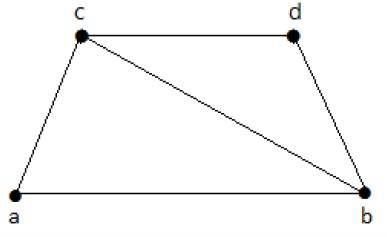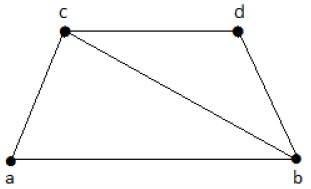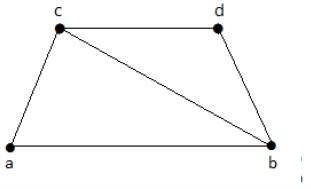# Graph Theory - Coverings

A covering graph is a subgraph which contains either all the vertices or all the edges corresponding to some other graph. A subgraph which contains all the vertices is called a line/edge covering. A subgraph which contains all the edges is called a vertex covering.

## Line Covering

Let G = (V, E) be a graph. A subset C(E) is called a line covering of G if every vertex of G is incident with at least one edge in C, i.e.,

deg(V) ≥ 1 ∀ V ∈ G

because each vertex is connected with another vertex by an edge. Hence it has a minimum degree of 1.

Example

Take a look at the following graph −Its subgraphs having line covering are as follows −

C1 = {{a, b}, {c, d}}

C2 = {{a, d}, {b, c}}

C3 = {{a, b}, {b, c}, {b, d}}

C4 = {{a, b}, {b, c}, {c, d}}

Line covering of ‘G’ does not exist if and only if ‘G’ has an isolated vertex. Line covering of a graph with ‘n’ vertices has at least [n/2] edges.

## Minimal Line Covering

A line covering C of a graph G is said to be minimal if no edge can be deleted from C.

Example

In the above graph, the subgraphs having line covering are as follows −

C1 = {{a, b}, {c, d}}

C2 = {{a, d}, {b, c}}

C3 = {{a, b}, {b, c}, {b, d}}

C4 = {{a, b}, {b, c}, {c, d}}

Here, C1, C2, C3 are minimal line coverings, while C4 is not because we can delete {b, c}.

## Minimum Line Covering

It is also known as Smallest Minimal Line Covering. A minimal line covering with minimum number of edges is called a minimum line covering of ‘G’. The number of edges in a minimum line covering in ‘G’ is called the line covering number of ‘G’ (α1).

Example

In the above example, C1 and C2 are the minimum line covering of G and α1 = 2.

• Every line covering contains a minimal line covering.

• Every line covering does not contain a minimum line covering (C3 does not contain any minimum line covering.

• No minimal line covering contains a cycle.

• If a line covering ‘C’ contains no paths of length 3 or more, then ‘C’ is a minimal line covering because all the components of ‘C’ are star graph and from a star graph, no edge can be deleted.

## Vertex Covering

Let ‘G’ = (V, E) be a graph. A subset K of V is called a vertex covering of ‘G’, if every edge of ‘G’ is incident with or covered by a vertex in ‘K’.

Example

Take a look at the following graph −The subgraphs that can be derived from the above graph are as follows −

K1 = {b, c}

K2 = {a, b, c}

K3 = {b, c, d}

K4 = {a, d}

Here, K1, K2, and K3 have vertex covering, whereas K4 does not have any vertex covering as it does not cover the edge {bc}.

## Minimal Vertex Covering

A vertex ‘K’ of graph ‘G’ is said to be minimal vertex covering if no vertex can be deleted from ‘K’.

Example

In the above graph, the subgraphs having vertex covering are as follows −

K1 = {b, c}

K2 = {a, b, c}

K3 = {b, c, d}

Here, K1 and K2 are minimal vertex coverings, whereas in K3, vertex ‘d’ can be deleted.

## Minimum Vertex Covering

It is also known as the smallest minimal vertex covering. A minimal vertex covering of graph ‘G’ with minimum number of vertices is called the minimum vertex covering.

The number of vertices in a minimum vertex covering of ‘G’ is called the vertex covering number of G (α2).

Example

In the following graph, the subgraphs having vertex covering are as follows −

K1 = {b, c}

K2 = {a, b, c}

K3 = {b, c, d}Here, K1 is a minimum vertex cover of G, as it has only two vertices. α2 = 2.